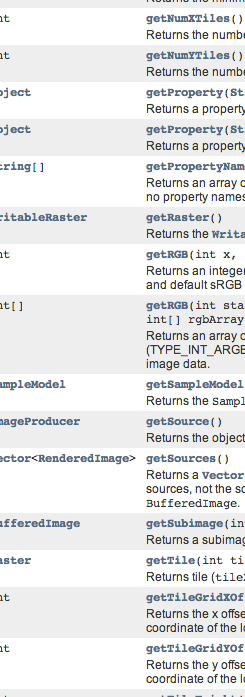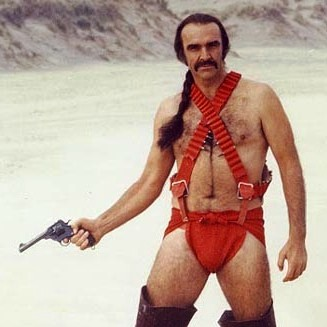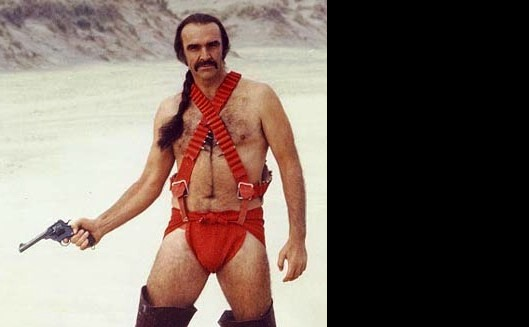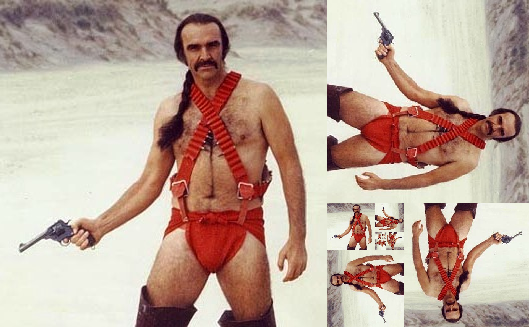# PureImage: raster image processing for Scala

## The problem

### They feature byzantive and opaque APIs### Mutable data structures

(I'm preaching to the choir here)

### Or require concrete representations of images

``````Image *ConstituteImage(
const size_t columns,
const size_t rows,
const char *map,
const StorageType storage,
const void *pixels,
ExceptionInfo *exception
)``````

## Images are simple things

### Let's give them a simple representation

``````trait Image[A] {
def width: Int
def height: Int
def apply(x: Int, y: Int):A
}``````

## PureImage

• Simple
• (Mostly) purely functional
• Fun

## parsing

``````package ps.tricerato.pureimage

case class RGBImage(image: Image[RGB]) extends LoadedImage
case class RGBAImage(image: Image[RGBA]) extends LoadedImage
case class GrayImage(image: Image[Gray]) extends LoadedImage

object Input {
}``````

## Colors

• Overall API is generic; we can represent a pixel as everything
• For import/export, we support three types of pixels.
• Internal represenation is as Ints:
``````case class RGB(i: Int) extends AnyVal {
def red = (i & 0xff)
def green = ((i >> 8) & 0xff)
def blue = ((i >> 16) & 0xff)
}
case class RGBA(i: Int) extends AnyVal {
def red = (i & 0xff).toByte
def green = ((i >> 8) & 0xff).toByte
def blue = ((i >> 16) & 0xff).toByte
def alpha = ((i >> 24) & 0xff).toByte
}
case class Gray(i: Int) extends AnyVal {
def white = (i & 0xff).toByte
}
``````
How can we work with pixels whose representation may be completely arbitrary in a generic way?

## Typeclasses

``````trait Pixel[A] {
def sum(a: A, b: A):A
def zero: A
}

def f[A : Pixel](image: Image[A]) = {
val ops = image.ops
...
}
``````

## Output

• Arbitrary number of pixel types
• Arbitrary number of image formats
• Many output formats don't support some pixel types (JPEG doesn't support an Alpha channel)
• The solution?

## Typeclasses

``````trait OutputFormat
case class JPEG(quality: Int) extends OutputFormat
object PNG extends OutputFormat
object GIF extends OutputFormat

trait Output[I, O <: OutputFormat] {
def apply(i: Image[I], o: O):Array[Byte]
}``````

## Let's try something...

First, we need a picture.
``````Input(resource("/zardoz.jpeg")) match {
case Right(RGBImage(image)) => image
case _ => ???
}``````This will do.``````def fib1[A : Pixel](image: Image[A]):Image[A] = {
import filter._
val square = squareCrop(image)
new Image[A] {
def width = (square.width * PHI).toInt; def height = square.height
def apply(x: Int, y: Int) = if (x >= square.width) {
image.ops.zero
} else {
image(x,y)
}
}
}````````````def fib2[A : Pixel](image: Image[A]):Image[A] = {
import filter._
val square = squareCrop(image)
new Image[A] {
def width = (square.width * PHI).toInt; def height = square.height
def apply(x: Int, y: Int) = {
val flipped = Rotate(Rotate.Clockwise90, image)
if (x >= square.width) {
flipped(x - square.width, y)
} else {
image(x,y)
}
}
}
}````````````def fib3[A : Pixel](image: Image[A]):Image[A] = {
import filter._
val square = squareCrop(image)
new Image[A] {
def width = (square.width * PHI).toInt; def height = square.height
def apply(x: Int, y: Int) = {
lazy val flipped = Rotate(Rotate.Clockwise90, fib3(image))
if (x >= square.width) {
flipped(x - square.width, y)
} else {
image(x,y)
}
}
}
}````````````def fib4[A : Pixel](image: Image[A]):Image[A] = {
import filter._
val square = squareCrop(image)
new Image[A] {
def width = (square.width * PHI).toInt; def height = square.height
def apply(x: Int, y: Int) = {
lazy val flipped = Rotate(Rotate.Clockwise90, fib4(image))
lazy val scaled = scale(
flipped.height.toFloat / image.height,
flipped
)
if (x >= square.width) {
scaled(x - square.width, y)
} else {
image(x,y)
}
}
}
}````````````def fib5[A : Pixel](image: Image[A]):Image[A] = {
import filter._
val square = squareCrop(image)
new Image[A] {
def width = (square.width * PHI).toInt; def height = square.height
def apply(x: Int, y: Int) = {
lazy val flipped = Rotate(Rotate.Clockwise90, fib4(image))
lazy val filtered = Lanczos(PHI.toFloat * 2)(flipped)
lazy val scaled = scale(flipped.height.toFloat / image.height, filtered)
if (x >= square.width) {
scaled(x - square.width, y)
} else {
image(x,y)
}
}
}
}
``````

## Included filters

• Lanczos
• Translate
• Scale
• Crop
• Alpha blending
• Whatever you want to write

## Lanczos filtering## Problems

### External image libraries

• Everything is terrible
• Java/AWT imaging: crazy and buggy
• Apache Imaging: slow
• C libraries: they are written in C

### Boxing#### In production, no single optimization proved as fruitful as avoiding boxing

• Image processing involves very many small calculations
• Allocation, incrementing heap pointers, and triggering (generational) GC aren't that expensive...
• But they're more expensive than bitwise operations of primitives on the stack
• Hence, boxing-related overhead can quickly dominate
• Generics work well
• Specialization works well
• Value classes work well
• They don't work well together [SI-5611]
``````clasee WrappedInt(val i: Int) extends AnyVal

def fooRaw(i: Int) = ??? // no boxing

def fooWrapped(w: WrappedInt) = ??? // no boxing

def fooGeneric[@specialized(Int) A](i: I) = ???
fooGeneric(42) // no boxing

fooGeneric(WrappedInt(42)) // boxing!
``````